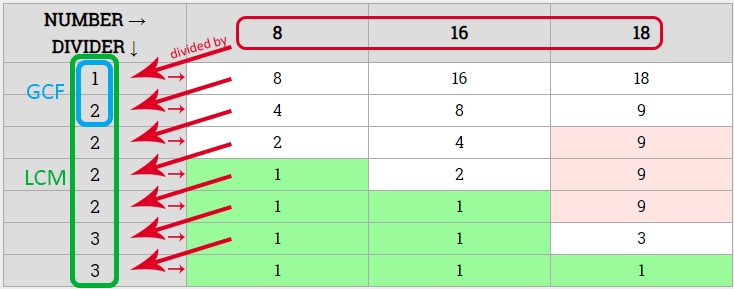## Friday, August 3, 2018

### Math: Finding GCF and LCM Using Continuous Division (Translated to JavaScript)

Hi, in this, I'll show a method other than factorisation and clustering the factors to find GCF (Greatest Common Factor) and LCM (Least Common Multiple).

I assume you're familiar with the first method. That is using tree factors and then grouping the dilly. I was taught to do this method in elementary school, maybe. The method has already been translated to an application on Port Raptor: GCFLCM.C. There's an explanation about the method flow on that post too.

Let's start to implement the continuous division method.

For example, we want to find GCF and LCM from

`8, 16, 18`

Method:

1. Create a table and write down those numbers as first row. Give some space for vertical column on the leftmost. The leftmost column will be used to list the divisor. The cells between the first row and first column are the division results (quotients). The division goes diagonally.
3. Then, divide starting from the lowest prime number → 2.
4. If an element (or elements) can't be divided with current prime divisor, but other element (or elements) can be divided, put the original element(s) which can't be divided to the next division result cell. Or, for element which can't be divided, leave it intact.
5. Increment the divisor to higher prime number if ALL division result can't be divided with current prime number.
6. Do it repetitively until the division result of each number is uniformly one (1).
7. For each row which ALL elements can be divided (no remainder), include the divisor(s) as GCF.
8. For each row which SOME (at least one) elements can be divided AND ALL elements can be divided, include the divisor(s) as LCM.

AS SUCH

NUMBER → DIVIDER ↓ 81618
1 81618
2 489
2 249
2 129
2 119
3 113
3 111

WITH MARKERSWHICH ONE IS GCF?

From the table above, look at the leftmost column, the divider (divisor). The ones which can divide ALL elements (numbers) are included as GCF.
Those are 1 and 2 → 1 × 2 = 2.

WHICH ONE IS LCM?

Look again at the divider (divisor) column, the ones which can divide SOME elements (at least one element) AND can divide ALL elements are included as LCM.
Basically, ALL numbers on the divisor column.
Those are 1, 2, 2, 2, 2, 3, and 3.
Multiply all of those → 1 × 2 × 2 × 2 × 2 × 3 × 3 = 144.

RESULT SUMMARY

Therefore, using the continuous division above:

For these numbers → `8, 16, 18`

GCF = 1 × 2 = `2`

LCM = 1 × 2 × 2 × 2 × 2 × 3 × 3 = `144`

As you can see from above example, it's very easy to determine the LCM → just multiply ALL the divisors.

Now, about the 1 divisor part. It is to accommodate GCF.
For instance: 2 and 3. Both are prime numbers. The GCF will be 1. LCM will be 1 × 2 × 3 = 6.
You can try it out using the table method above.

MORE EFFICIENT

This is pretty much a direct method, rather than using tree factors and grouping/clustering. It's way more efficient and we can put as many numbers as we need to calculate on the table.

TRANSLATION

I first found this from a school textbook. So then, I translated it to JavaScript. The JavaScruot, wut, JavaScript coding can also be translated to other languages surely.

I made a flowchart of the method. I hope the chart explains the flow.

Thanks to draw.io for the flowchart.I'm not sure if that flowchart is 100% accurate. But that looks like the basic concept.

JAVASCRIPT FUNCTION

The function name is `glc`

It receives an argument. That is an array of positive integers, which each is greater or equal to 2, to 1,000,000 (1 million).

The minimum array length is 2, maximum is 20.

Code is available on MR's Github reposityro

HOW TO USE

Run:

Example:

ARRAY INPUT

Input must be an array of positive integers with minimum array length of 2 and maximum length of 20.

Each array item must be below 1,000,000 (1 million).

OUTPUT

For valid input, the output will be an `object type` consists of:

For example:

For invalid input, the output will be error string, `string type`.

ACCESSING PARTICULAR OUTPUT'S ITEM

First of all, check if the calculation output is an `object` before you do this.
To check whether it's an `object`

Interface

Thanks to codepen.io

See the Pen Itnerfce for something by Monkey Raptor (@monkeyraptor) on CodePen.

Thanks for stopping by, and bye, see you again.